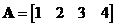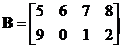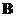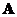## Matrix Vertical Concatenation

Matrix vertical concatenation is an operation to join two sub matrices vertically into one matrix.

ExampleVertical concatenation of the two matrices is adjoining matrixto matrixto produce augmented matrixWhen we concatenate vertically, the number of columns of the two input matrices must be the equal.

The interactive program below produces vertical concatenation of the two input matrices. Your input matrices must have the same number of columns. Random Example will generate random matrices at the proper size. Experiment with your own input matrices to gain more understanding about matrix augmentation.

;

See also: matrix horizontal concatenation